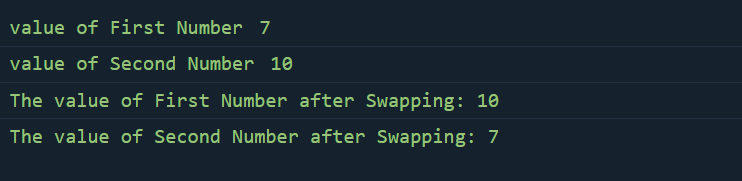top of page
Search

# One-Liner code in JavaScriptJavaScript syntax and built-in methods allows you to cut down a lot of unnecessary lines in your code and write short, easily readable code. As we all know less code is performs faster and better. it’s also easy understand, remember and maintain.

1. Check if Data is weekend or Not?

2. Even or Odd Number

3. Unique value in array

4. Generate random number between two numbers

5. Swapping two variables

### 1. Check if Date is weekend or Not?

This program is to determine if the given date is weekend (we will consider Saturday and Sunday as weekend). We will use .getDay() method on the Date object to get the day.

##### Code:
```function isWeekend(date = new Date()) {
return date.getDay() % 6 === 0;
}

const d1 = new Date('2022-08-22');
console.log(d1); //

console.log(isWeekend(d1));

const d2 = new Date('2022-02-20');
console.log(d2);

console.log(isWeekend(d2));```

##### Output:### 2. Even or Odd Number

In this, X % 2 == 0 checks whether the number is even. If the remainder is 0 then the number is even and if the remainder is 1 then the number is Odd.

##### Code:
```var x = 11;
console.log("The given number is:",x);
if (x % 2 ==0)
{
Console.log("The number is even");
}
else
{
console.log("The number is odd");
}```

##### Output:In the above example, when the number 11 is divided by 2, the remainder is 1. So the result will be "The number is Odd"

### 3. Unique value in array

We have an array that contains duplicate elements like this −

`const arr = [1,2,2,3,3,4,4,5];`

We will write the Javascript function which displays the above array and return new array with unique value.

The array will only contain the elements that only appear once in the original array.

##### Code:
```const arr = [1,2,2,3,3,4,4,5];
const extractUnique = arr => {
const res = [];
for(let i = 0; i < arr.length; i++){
if(arr.lastIndexOf(arr[i]) !== arr.indexOf(arr[i])){
continue;
};
res.push(arr[i]);
};
return res;
};
console.log("Array list:", arr);
console.log("Unique Values in the list are:",extractUnique(arr));```

##### Output:### 4. Generate Random Numbers between two Numbers

The Math.random() function returns a floating-point, pseudo-random number in the range 0 to less than 1 (inclusive of 0, but not 1) with approximately uniform distribution over that range — which you can then scale to your desired range. The implementation selects the initial seed to the random number generation algorithm; it cannot be chosen or reset by the user.

##### Code:
```function getRandomInt(max) {
return Math.floor(Math.random() * max);
}

console.log(getRandomInt(3));
// expected output: 0, 1 or 2

console.log(getRandomInt(1));
// expected output: 0

console.log(Math.random());
// expected output: a number from 0 to <1```

##### Output:### 5. Swapping two variables

Here we swap two variables using temp number which is used to hold the value of one of the variable, say a. The array value which we let the temporary variable hold is reassigned by the second array value(b). Finally, b(second variable) is given the value of temp which is a.

##### Code:
```var num1 = prompt('Enter first number: ');
var num2 = prompt('Enter second number: ');
console.log("value of First Number", num1);
console.log("value of Second Number", num2);

// Temporary variable
var temp;

// Swapping two variables
temp = num1;
num1 = num2;
num2 = temp;

// Displaying output
console.log('The value of First Number after Swapping: ' + num1);
console.log('The value of Second Number after Swapping: ' + num2);```

##### Output:The Tech Platform